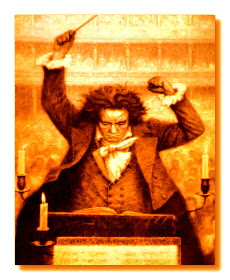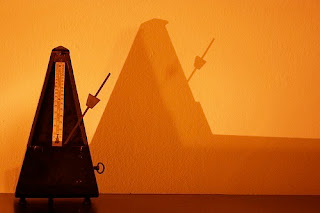# Metronome Marks

A metronome is a chronometric (i.e., a time measuring) device designed to perform two main functions: to signal the passing of each unit of time with a clicking sound, and to mathematically scale time.
Contrary to the functioning of a regular clock which invariably outputs 60 seconds per minute, the metronome's mechanism assumes that rate of units, not as a constant, but rather as a user assigned variable. By choosing a different rate than the referential 60 seconds per minute, the user is in effect scaling time.Beethoven conducting.

It took just a few years after its commercial release for the metronome to become a referential instrument among musicians. By 1817, Beethoven was using a  metronome model by Johann Mälzel (1772–1838) and, for the first time, documented metronome marks as means to define tempo in his works.
Mälzel's metronome featured a clockwork mechanism inside a pyramid-shaped wooden box. Outside the box, a metal weight on a calibrated pendulum rod. While the pendulum swinged back and forth at a constant rate (the lower the weight, the faster the pendulum swings, and vice versa), the inside mechanism produced a clicking sound, ear-marking each oscillation. Adjusting the rate of clicks per second was done by simply sliding the weight up or down the pendulum. The pendulum rod came preset with a finite number of metronome marks, leaving the desired rate as a choice of one from a logarithmic scale of pre-defined tempo rates. On  mechanical metronomes, tempo marks range between a rate of 40 to 208 units per minute. With the advent of digital instruments, any positive integer or floating point number can be used to scale time.Mälzel's Metronome

Defining a metronome mark  requires a minimum of two parameters:  a time-unit (typically represented by a note-value), and a number for the respective rate of time-units per minute (a.k.a., Beats Per Minute - BPM). Other standard representations of metronome marks include using the equal sign " = " between the two parameters above, and less frequently, to have metronome marks proceeded by the initials MM, for Mälzel's Metronome.